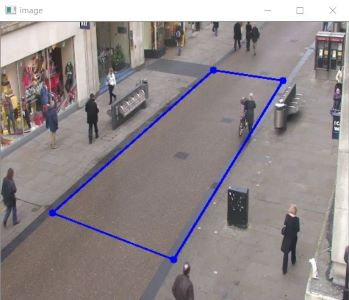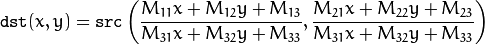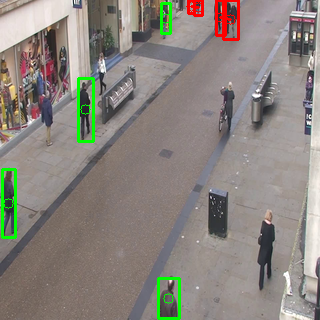# Real Time Social Distance Detector

The report explains how to build a fast and accurate social distance detector to run on-device inference using real-time video feed.
Piyush Thakur## Introduction

### Check out the Colab Notebook

In any pandemic where the disease spreads through physical contact, social distancing has always been the most efficient method to counteract the disease. So, in recent times where Covid-19 has devastated the lives of many, maintaining social distance is essential. A majority of industries are demanding a tool to determine the extent to which people are following this safety protocol.
A social distance detector is one such way to address this kind of situation.

## Approach

To serve this purpose, there are three main aspects of the pipeline :
1. Real Time Pedestrian Detection : Object Detection is a combination of object classification and object localization. It is trained to detect the presence and location of multiple classes of objects. Here, the object which we are addressing is 'human'. We need to identify the particular class, i.e. people, in real-time video feed with sufficient confidence. There are various approaches for object detection - it can be Region Classification Method with R-CNN or Fast R-CNN, Implicit Anchor Method with Faster R-CNN, YOLO v1-v4, or EfficientDet, Keypoint Estimation Method with CenterNet or CornerNet.
Our motto is to detect pedestrians from a scene and locate their coordinates. For on-device inference, we will use SSD(Single Shot Detector) model. This model will be converted to TensorFlow Lite from TensorFlow Lite Object Detection Api(TFOD). The mobile model used here is SSD_MobileDet_cpu_coco.
1. Calibration : Each frame will have an arbitrary perspective view, which causes difficulty to analyze or calculate uniformly the relative distance between the people. So, it’s necessary to convert the frame view into a bird's eye (top-down) view. This process is termed as calibration. This ensures that everyone is on the same flat ground plane. This is a step to improve our Social-distance Detector and yield accurate measurements in the final phase of pipeline.
2. Determining social distance violation : Now that we have positions of people in the top-down view, the final step of the pipeline is to check if any person is in close proximity to one or more people. People following the norm are welcomed with a green bounding box, while the violators are highlighted with the red one with two or more connecting lines.
The following will be discussed :
• TensorFlow Lite and MobileDet
• Model Conversion
• Model Benchmarks for MobileDet variants
• Calibration and Transformation
• Determining social distance violation
• Visualization
• Inference
• Conclusion

## TensorFlow Lite and MobileDet

Talking of on-device inference, MobileDet serves the best. On the COCO object detection task, MobileDets outperform MobileNetV3+SSDLite by 1.7 mAP at comparable mobile CPU inference latencies. MobileDets also outperform MobileNetV2+SSDLite by 1.9 mAP on mobile CPUs. You can know more about MobileDet from here
Converting this model to TensorFlow Lite has a purpose as it enables on-device machine learning inference with low latency and a small binary size. TensorFlow Lite is build for developers to run TensorFlow models on mobile, embedded and IoT devices. You can learn more about TensorFlow Lite from here
SSD(Single Shot Detector) model takes an image as input. Let's consider, if the input image is of 300x300 pixels, with three channels (red, blue, and green) per pixel, which is then fed to the model as a flattened buffer of 270,000 byte values (300x300x3). If the model is quantized, each value should be a single byte representing a value between 0 and 255. This model outputs 4 arrays(Location, Classes, Confidences, Number of Detection). If we have to convert this model to TensorFlow Lite, we have to first generate the frozen graph that is compatible with the TensorFlow Lite operator set(as explained here - TF1 or TF2). The two scripts(TF1 and TF2) add optimized postprocessing to the model graph. This model graph is later on quantized to TensorFlow Lite model file with .tflite extension with three quantization process.
In the next section, we will see these three quantization process :
1. Dynamic Range quantization
2. Float 16 quantization
3. Full Integer quantization

## Model Conversion

This is the model SSD_MobileDet_cpu_coco which we will quantize ahead. When this model bundle is untar'd, we get following files : pre-trained checkpoints, a TensorFlow Lite (TFLite) compatible model graph, a TFLite model file, a configuration file, and a graph proto. The models were pre-trained on the COCO dataset. model.ckpt-* files are the pre-trained checkpoints on the COCO dataset. The tflite_graph.pb file is a frozen inference graph that is compatible with the TFLite operator set, which was exported from the pre-trained model checkpoints. model.tflite file is a TFLite model that was converted from the tflite_graph.pb frozen graph.
`--- model.ckpt-400000.data-00000-of-00001--- model.ckpt-400000.index--- model.ckpt-400000.meta--- model.tflite--- pipeline.config--- tflite_graph.pb--- tflite_graph.pbtxt`

#### Dynamic Range Quantization

`converter = tf.compat.v1.lite.TFLiteConverter.from_frozen_graph( graph_def_file=model_to_be_quantized, input_arrays=['normalized_input_image_tensor'], output_arrays=['TFLite_Detection_PostProcess','TFLite_Detection_PostProcess:1','TFLite_Detection_PostProcess:2','TFLite_Detection_PostProcess:3'], input_shapes={'normalized_input_image_tensor': [1, 320, 320, 3]})converter.allow_custom_ops = Trueconverter.optimizations = [tf.lite.Optimize.DEFAULT]tflite_model = converter.convert()`
This dynamic range quantizes the weights from floating point to integer, which has 8-bits of precision. At inference, weights are converted from 8-bits of precision to floating point and computed using floating-point kernels. In the code block above, we need to give the tflite_graph.pb file in place of model_to_be_quantized. The model accepts the input image to be of 320*320 pixels, so the input_arrays and input_shapes are set according to that. The output_arrays are set to output four arrays : Location of bounding box, Classes of object detected, Confidences, Number of Detections. These are set according to this guide
After quantization, we get a model size of 4.9MB.

#### Float 16 Quantization

`converter = tf.compat.v1.lite.TFLiteConverter.from_frozen_graph( graph_def_file=model_to_be_quantized, input_arrays=['normalized_input_image_tensor'], output_arrays=['TFLite_Detection_PostProcess','TFLite_Detection_PostProcess:1','TFLite_Detection_PostProcess:2','TFLite_Detection_PostProcess:3'], input_shapes={'normalized_input_image_tensor': [1, 320, 320, 3]})converter.allow_custom_ops = Trueconverter.target_spec.supported_types = [tf.float16]converter.optimizations = [tf.lite.Optimize.DEFAULT]tflite_model = converter.convert()`
This float 16 quantization reduces the model size to half with minimal loss in accuracy. It quantizes model weights and bias values from full precision floating point (32-bit) to a reduced precision floating point data type (IEEE FP16). We just have to add one line of code to the previous dynamic range code block : converter.target_spec.supported_types = [tf.float16]
After quantization, we get a model size of 8.2MB.

#### Full Intezer Quantization

`converter = tf.compat.v1.lite.TFLiteConverter.from_frozen_graph( graph_def_file=model_to_be_quantized, input_arrays=['normalized_input_image_tensor'], output_arrays=['TFLite_Detection_PostProcess','TFLite_Detection_PostProcess:1','TFLite_Detection_PostProcess:2','TFLite_Detection_PostProcess:3'], input_shapes={'normalized_input_image_tensor': [1, 320, 320, 3]})converter.allow_custom_ops = Trueconverter.representative_dataset = representative_dataset_genconverter.inference_input_type = tf.uint8converter.quantized_input_stats = {"normalized_input_image_tensor": (128, 128)}converter.optimizations = [tf.lite.Optimize.DEFAULT]tflite_model = converter.convert()`
For Full Integer Quantization Method, we need to use the representative dataset. To make your own dataset, you can look over here. The function to generate representative dataset is shown here. This dataset can be a small subset around 100-150 samples of training or validation data. Representative Dataset is needed to calibrate the variable tensors such as model input, activations(outputs of intermediate layers) and model output by running a few inference cycles.
After quantization, we get a model size of 4.9MB.

## Model Benchmarks for MobileDet variants

Model Benchmark is a way of choosing the best model for your purpose. One way is to know by looking at their FPS and Elapsed Time. Here are some of the model benchmarks I recorded :
Model Name Model Size(MB) Elapsed Time(s) FPS
SSD_mobileDet_cpu_coco_int8 4.9 705.32 0.75
SSD_mobileDet_cpu_coco_fp16 8.2 52.79 10.06
SSD_mobileDet_cpu_coco_dr 4.9 708.57 0.75

One more way is to use the TensorFlow Lite Benchmark Tool. You have to configure Android Debug Bridge(adb) in your laptop and connect it with your android device to use TensorFlow Lite Benchmark Tool and check the inference speed of the model. I have shown only the fp16 one as this is the fastest among all the three variants.
• The first result in the image given below is with the use of cpu with 4 threads.
• The second result in the image given below is with the use of gpu.## CalibrationThe sequence of steps involve:

#### 1. Finding a quadrilateral - 4 corner points

Transforming the image frame from perspective to top-down (or bird's eye) view is possible on the basis of selection of right coordinates of a quadrilateral, upon which the image frame is warped. For this, the targets can be street lanes, floor or ground.
Calibration is solely based on the background in an image. So, if background view changes, quad-points changes, and so changes transformation matrix to calibrate.
Assuming that the camera position is fixed and so the background, the easiest way is to find 4 corner-points (of road or floor) on the ground in the pre-processing step. As of now, we have done so using user-interactive mouse click operation of mouse_click_event.py that allows user to select a quadrilateral by drag-and-drop of corners.#### 2. Transformation matrix (M)

Transformation matrix is the main source for calibration.
After fetching the chosen corner points, order the coordinates and apply getPerspectiveTransform() of OpenCV to get the transformation matrix (M). In order to warp the entire image frame, use the mapping function :where (x,y) refers to the source image coordinates.
Apply this function to map the image corner points (not the ones from mouse click!) and get the corresponding coordinates in top-down view. Then, evaluate its extreme coordinates (extreme left, right, top, bottom points in the warped frame) and utilize them to get the final transformation matrix M.
`def getmap(image): # image : first frame of the input video global grid_H, grid_W h,w=image.shape[:2] # 4 corner points of image are set by default # User needs to finalise the corner points of a road or floor by dragging and dropping the corners #corners=mouse_click_event.adjust_coor_quad(image,corners) corners=[(308, 67), (413, 91), (245, 351), (75, 270)] corners=np.array(corners,dtype="float32") src=order_points(corners) (tl,tr,br,bl)=src width1=np.sqrt(((br-bl)**2)+((br-bl)**2)) width2=np.sqrt(((tr-tl)**2)+((tr-tl)**2)) width=max(int(width1),int(width2)) height1=np.sqrt(((tr-br)**2)+((tr-br)**2)) height2=np.sqrt(((tl-bl)**2)+((tl-bl)**2)) height=max(int(height1),int(height2)) width=int(width) height=int(height) dest=np.array([[0,0],[width-1,0],[width-1,height-1],[0,height-1]],dtype="float32") M=cv2.getPerspectiveTransform(src,dest) corners=np.array([[0,h-1,1],[w-1,h-1,1],[w-1,0,1],[0,0,1]],dtype="int") warped_corners=np.dot(corners,M.T) warped_corners=warped_corners/warped_corners[:,2].reshape((len(corners),1)) warped_corners=np.int64(warped_corners) warped_corners=warped_corners[:,:2] min_coor=np.min(warped_corners,axis=0) max_coor=np.max(warped_corners,axis=0) grid_W,grid_H=max_coor-min_coor dest=np.array([[abs(min_coor),abs(min_coor)],[abs(min_coor)+grid_W-1,abs(min_coor)],[abs(min_coor)+grid_W-1,abs(min_coor)+grid_H-1],[abs(min_coor),abs(min_coor)+grid_H-1]],dtype="float32") M=cv2.getPerspectiveTransform(src,dest) return M`
As discussed before, getmap() is supposed to be called only once in the pre-processing step so as to get the transformation matrix and calibrate points in every frame.

#### 3. Calibrate

Using the transformation matrix, we need to:
1. calibrate the positions of detected people to their corresponding positions in the warped image or "bird's eye view grid".
2. calculate the minimum social distance to be maintained - MIN_DISTANCE.
An average person height is 5-6 feet. And we need to maintain social distance of 6 feet. Thus, from the results of pedestrian detector, the median height of people is determined, and we set this height as MIN_DISTANCE. But, this height needs to be calibrated in order to use it for Distance-Violation Determination (which is completely based on top-down view).
`def calibration(M,results): # calculate minimum distance for social distancing global MIN_DISTANCE rect=np.array([r for r in results]) h=np.median(rect[:,2]-rect[:,0]) coor=np.array([[50,100,1],[50,100+h,1]],dtype="int") coor=np.dot(coor,M.T) coor=coor/coor[:,2].reshape((2,1)) coor=np.int64(coor[:,:2]) MIN_DISTANCE=int(round(dist.pdist(coor))) #calculate centroid points of detected people location corresponding to bird's eye view black grid centroids=np.array([r for r in results]) centroids=np.c_[centroids,np.ones((centroids.shape,1),dtype="int")] warped_centroids=np.dot(centroids,M.T) warped_centroids=warped_centroids/warped_centroids[:,2].reshape((len(centroids),1)) warped_centroids=np.int64(warped_centroids) return warped_centroids[:,:2]`## Determining Social Distance Violation

To calculate distance between people, the brute force method is to evaluate the Euclidean distances between every possible pair and determine which pair(s) crosses the limit. This can be easily achieved by utilizing the fast and advanced python libraries - numpy and scipy. Check this out to know more.`def calc_dist(centroids): # centroids : updated centroids in top-down view coordinates if len(centroids)<2: # no pair of people, no violation return list() # evaluate the pairwise distances between people condensed_dist=dist.pdist(centroids) D=dist.squareform(condensed_dist) locations=np.where(D`

## `Visualization`

`Everything is useless without visualizing what's happening!`
`In our project, color "green" symbolizes people following social distancing and color "red" remarks people violating the safety norm.`
`Basically, the following frames are used for visualization:`
1. `Output - shows the input video frames in addition to green and red bounding boxes around the people. It also shows red lines connecting the violators for better understanding.`
2. `Bird's eye view grid - shows a black frame locating all the people in top-down view with their color codes (green/red) as points. One or more red lines are connected between the points when the points (or people) come in close proximity.`
3. `Warped image - (optional, for understanding operations) shows the top-down view of input video frames with the color-coded points on people.`

#### `Visualize the output frame`

`The results of pedestrian detector helps to locate people. The list of violators helps to segregate the two class of people. Draw the bounding boxes choosing colors as per specified convention.`
`def visualise_main(frame,results,violate): for (i,(prob,bbox,centroid)) in enumerate(results): (startX,startY,endX,endY)=bbox (cX,cY)=centroid colour=(0,255,0) if i in np.unique(violate): colour=(0,0,255) frame=cv2.rectangle(frame,(startX,startY),(endX,endY),colour,2) frame=cv2.circle(frame,(cX,cY),5,colour,1) #Drawing the connecting lines between violators for i,j in violate: frame=cv2.line(frame,results[i],results[j],(0,0,255),2) return frame`
``

#### `Visualize Bird's eye view grid and Warped Frames`

`Get the calibrated warped image of the main frame using cv2.warpPerspective().`
``
`From calibrated data, locate people in bird's eye view and put them as dotted points in a black grid. Color is chosen in accordance with the list of violators. For all pairs of violators, draw a red line between them.`
``
`def visualise_grid(image,M,centroids,violate): warped=cv2.warpPerspective(image,M,(grid_W,grid_H),cv2.INTER_AREA, borderMode=cv2.BORDER_CONSTANT, borderValue=(0,0,0)) grid=np.zeros(warped.shape,dtype=np.uint8) for i in range(len(centroids)): colour=(0,255,0) if i in np.unique(violate): colour=(0,0,255) grid=cv2.circle(grid,tuple(centroids[i,:]),5,colour,-1) warped=cv2.circle(warped,tuple(centroids[i,:]),5,colour,-1) for i,j in violate: grid=cv2.line(grid,tuple(centroids[i]),tuple(centroids[j]),(0,0,255),2) #cv2_imshow("Bird's eye view grid",cv2.resize(grid,image.shape[:2][::-1])) #cv2_imshow("warped",cv2.resize(warped,image.shape[:2][::-1])) grid=cv2.resize(grid,image.shape[:2][::-1]) warped=cv2.resize(warped,image.shape[:2][::-1]) return grid,warped`

## `Inference`

`For running TensorFlow Lite model on-device, so that it could make predictions based on input data. This process is Inference. For inference, we need to run it through an interpreter. TensorFlow Lite inference follow these steps given below :`

#### `Load the model`

`As SSD_MobileDet_cpu_coco_fp16 showed the best result among all three, we will be going on with loading this model. The tf.lite.Interpreter takes in the .tflite model file. The tensors are allocated and the model input shape is defined in HEIGHT, WIDTH.`
`tflite_model = "ssd_mobiledet_cpu_coco_fp16.tflite" interpreter = tf.lite.Interpreter(model_path=tflite_model)interpreter.allocate_tensors()_, HEIGHT, WIDTH, _ = interpreter.get_input_details()['shape']`

#### `Set Input Tensor`

`The code block below will return all the input details of the model.`
`def set_input_tensor(interpreter, image): tensor_index = interpreter.get_input_details()['index'] input_tensor = interpreter.tensor(tensor_index)() input_tensor[:, :] = image`

#### `Get Output Tensor`

`The code block below will return all the output details : Location of Bounding Box, Class, Confidence, Number of detection.`
`def get_output_tensor(interpreter, index): output_details = interpreter.get_output_details()[index] tensor = np.squeeze(interpreter.get_tensor(output_details['index'])) return tensor`

#### `Pedestrian Detection`

`def pedestrian_detector(interpreter, image, threshold): """Returns a list of detection results, each as a tuple of object info.""" H,W=HEIGHT,WIDTH set_input_tensor(interpreter, image) interpreter.invoke() # Get all output details boxes = get_output_tensor(interpreter, 0) class_id = get_output_tensor(interpreter, 1) scores = get_output_tensor(interpreter, 2) count = int(get_output_tensor(interpreter, 3)) results = [] for i in range(count): if class_id[i] == 0 and scores[i] >= threshold: [ymin,xmin,ymax,xmax]=boxes[i] (left, right, top, bottom) = (int(xmin * W), int(xmax * W), int(ymin * H), int(ymax * H)) area=(right-left+1)*(bottom-top+1) if area>=1500: continue centerX=left+int((right-left)/2) centerY=top+int((bottom-top)/2) results.append((scores[i],(left,top,right,bottom),(centerX,centerY))) return results`

#### `Preprocessing Video Frames`

`Before running inference on videos, we need to preprocess all the video frames. The frames are resized to the accepted HEIGHT and WEIGHT of the model input image. Later on, the preprocessed image is converted to numpy array. It is most common to use 32-bit precision when training a neural network, so at one point we have to convert the input data to 32 bit floats. It's divided by 255, this is the maximum value of a byte (the input frames type before the conversion to float32), so this will ensure that the input frames are scaled between 0.0 and 1.0.`
`def preprocess_frame(frame): frame = Image.fromarray(frame) preprocessed_image = frame.resize( ( HEIGHT, WIDTH ), Image.ANTIALIAS) preprocessed_image = tf.keras.preprocessing.image.img_to_array(preprocessed_image) preprocessed_image = preprocessed_image.astype('float32') / 255.0 preprocessed_image = np.expand_dims(preprocessed_image, axis=0) return preprocessed_image`

#### `Output Video Generation Utils`

`Finally, all the required functions are applied sequentially. The detector is now in action.`
`def process(video): vs=cv2.VideoCapture(video) #Capture the first frame of video res,image=vs.read() if image is None: return image=cv2.resize(image,(320,320)) # get transformation matrix mat=getmap(image) fourcc=cv2.VideoWriter_fourcc(*"XVID") out=cv2.VideoWriter("result.avi",fourcc,20.0,(320*3,320)) fps = FPS().start() while True: res,image=vs.read() if image is None: break #pedestrian detection preprocessed_frame = preprocess_frame(image) results = pedestrian_detector(interpreter, preprocessed_frame, threshold=0.25) preprocessed_frame = np.squeeze(preprocessed_frame) * 255.0 preprocessed_frame = preprocessed_frame.clip(0, 255) preprocessed_frame = preprocessed_frame.squeeze() image = np.uint8(preprocessed_frame) #calibration warped_centroids=calibration(mat, results) #Distance-Violation Determination violate=calc_dist(warped_centroids) #Visualise grid grid,warped=visualise_grid(image,mat,warped_centroids,violate) #Visualise main frame image=visualise_main(image,results,violate) #Creating final output frame output=cv2.hconcat((image,warped)) output=cv2.hconcat((output,grid)) out.write(output) fps.update() fps.stop() print("[INFO] elapsed time: {:.2f}".format(fps.elapsed())) print("[INFO] approx. FPS: {:.2f}".format(fps.fps())) # release the file pointers print("[INFO] cleaning up...") vs.release() out.release()`

## `Conclusion`

`Our social distance developer runs successfully with an optimal throughput as :`
`[INFO] elapsed time: 52.79[INFO] approx. FPS: 10.06[INFO] cleaning up...`
`Further, the results are very fascinating - it shows results accurately in the runtime. Check it out here.`
``` window.__WB_SEO_BREADCRUMBS = [{"name":"Fully Connected","path":"/fully-connected"},{"name":"Keras or TensorFlow","path":"/fully-connected/keras-or-tensorflow"},{"name":"Real Time Social Distance Detector","path":"/social-distance-detector/real-time-social-distance-detector/reports/Real-Time-Social-Distance-Detector--Vmlldzo0Njc3OTI"}]; window.insertScript=function(n){return new Promise((e,r)=>{var o=document.createElement("script");o.src=n,o.async=!0,o.onload=e,o.onerror=r,document.body.appendChild(o)})}window.Prism=window.Prism||{highlightAll:function(){}}!function(){function e(e,n,t){Optanon.InsertScript(e,"body",t,null,n)}function n(){analytics.initialize||(analytics.SNIPPET_VERSION="4.0.0",e("https://cdn.wandb.ai/production/2951f9d4b803c6427417a051fbcd72604d0bd0f1/sa.min.js",2,()=>{null==window.FS||null==window.FS.shutdown||window.dontShutdownFS||window.FS.shutdown()}),e("https://cdn.wandb.ai/production/2951f9d4b803c6427417a051fbcd72604d0bd0f1/autotrack.js",2),window.ga=window.ga||function(){window.ga.q=window.ga.q||[],window.ga.q.push(arguments)},window.ga("require","eventTracker"),window.ga("require","outboundLinkTracker"),window.ga("require","urlChangeTracker"),window.ga("require","cleanUrlTracker"),window.ga("require","impressionTracker"),window.ga("require","maxScrollTracker"),window.ga("require","mediaQueryTracker"),window.ga("require","outboundFormTracker"),window.ga("require","pageVisibilityTracker"))}!function(){var e=window.analytics=window.analytics||[];if(e.invoked)console.error("Segment snippet included twice.");else{e.invoked=!0,e.methods=["trackSubmit","trackClick","trackLink","trackForm","pageview","identify","reset","group","track","ready","alias","debug","page","once","off","on","use","init"],e.factory=function(n){return function(){var t=Array.prototype.slice.call(arguments);return t.unshift(n),e.push(t),e}};for(var n=0;n<e.methods.length;n++){var t=e.methods[n];e[t]=e.factory(t)}}}(),window.analyticsOK=!(window.CONFIG&&window.CONFIG.DISABLE_TELEMETRY);const t=window.CONFIG&&window.CONFIG.ANALYTICS_DISABLED;window.thirdPartyAnalyticsOK=!t,t||window.initializedScripts||(insertScript("https://code.jquery.com/jquery-3.6.0.min.js").then(()=>insertScript("https://cdn.cookielaw.org/consent/da0f7ab4-835a-42da-beeb-767dcff7d1cc.js")),window.thirdPartyAnalyticsOK=!1,window.OptanonWrapper=function(){window.thirdPartyAnalyticsOK=!0,function(){!function(e,n,t,i,o){e[i]=e[i]||[],e[i].push({"gtm.start":(new Date).getTime(),event:"gtm.js"});var r=n.getElementsByTagName(t),a=n.createElement(t);a.async=!0,a.src="https://www.googletagmanager.com/gtm.js?id=GTM-TCTWFHR",r.parentNode.insertBefore(a,r)}(window,document,"script","dataLayer");const e=document.createElement("noscript");e.innerText='<iframe src="https://www.googletagmanager.com/ns.html?id=GTM-TCTWFHR" height="0" width="0" style="display:none;visibility:hidden"></iframe>',document.body.appendChild(e)}(),n(),function(){const n=/kaggle\.com/.test(window.location.host),t=/colab\.research\.google\.com/.test(window.location.host),i=/iPhone|iPad|Android/.test(navigator.userAgent);if(n||t||i)return;const o="https://static.zdassets.com/ekr/snippet.js?key=a0a3439e-c6f9-4864-8d97-4bc7bef38531";e(o,2,()=>{const e=document.querySelector(`script[src="\${o}"]`);if(null!=e){const n=document.createElement("div");n.id="zendesk-launcher",n.className="wbic-ic-chat-fill",n.onclick=()=>openWidget();const t=document.createElement("script");t.type="text/javascript",t.innerHTML="zE('webWidget', 'hide')\nfunction openWidget() {\n zE('webWidget', 'show');\n zE('webWidget', 'open');\n document.querySelector('#zendesk-launcher').style.display = 'none';\n}\nzE('webWidget:on', 'close', function() {\n zE('webWidget', 'hide');\n document.querySelector('#zendesk-launcher').style.display = 'block'\n})",e.after(n,t)}});const r=document.querySelector(`script[src="\${o}"]`);null!=r&&(r.id="ze-snippet")}(),e("https://ws.zoominfo.com/pixel/qhXboAuxB56YVRvedMdX",2),window.beamer_config={product_id:"iTpiKrhl12143",lazy:!0},e("https://app.getbeamer.com/js/beamer-embed.js",2)}),window.initializedScripts=!0}()!function(e){function r(r){for(var n,c,f=r,d=r,u=r,l=0,b=[];l<f.length;l++)c=f[l],Object.prototype.hasOwnProperty.call(a,c)&&a[c]&&b.push(a[c]),a[c]=0;for(n in d)Object.prototype.hasOwnProperty.call(d,n)&&(e[n]=d[n]);for(i&&i(r);b.length;)b.shift()();return o.push.apply(o,u||[]),t()}function t(){for(var e,r=0;r<o.length;r++){for(var t=o[r],n=!0,f=1;f<t.length;f++){var d=t[f];0!==a[d]&&(n=!1)}n&&(o.splice(r--,1),e=c(c.s=t))}return e}var n={},a={6:0},o=[];function c(r){if(n[r])return n[r].exports;var t=n[r]={i:r,l:!1,exports:{}};return e[r].call(t.exports,t,t.exports,c),t.l=!0,t.exports}c.e=function(e){var r=[],t=a[e];if(0!==t)if(t)r.push(t);else{var n=new Promise((function(r,n){t=a[e]=[r,n]}));r.push(t=n);var o,f=document.createElement("script");f.charset="utf-8",f.timeout=120,c.nc&&f.setAttribute("nonce",c.nc),f.src=function(e){return c.p+"static/js/"+({}[e]||e)+"."+{0:"68d61512",1:"341e2135",2:"754885d1",3:"6ef17cfc",4:"29e5c67e",8:"9f131731",9:"3eb2f79f",10:"12b7e9af",11:"72912378",12:"a39c6189",13:"d4429e9e",14:"9b855f9c",15:"b34f9fd2",16:"c4222200",17:"39f66019",18:"4050b006",19:"b6838460",20:"48c2c06d",21:"e70bc681",22:"a68d36af",23:"5075a4d0",24:"d08aed0f",25:"d12c800c",26:"ffb81bdc",27:"42758164",28:"cb39ed14",29:"7d08e891",30:"46ac8217",31:"30e7dc13",32:"5f7b2350",33:"05a97120",34:"d721cbc1",35:"66461ef8",36:"a28508c9",37:"df2d3d82",38:"e3e2d862",39:"dae907ef",40:"89345d91",41:"daf5f7c4",42:"929393a9",43:"1d1075ec",44:"38950c54",45:"22493aa1",46:"60e307b4",47:"5ef7c177",48:"d8d69f20",49:"c4af67f3",50:"a26bbc8e",51:"e01d9bad",52:"5f086e25",53:"9e752fe4",54:"b7baa7f9",55:"7c0827a7",56:"94ae29ac",57:"0b61d752",58:"2d1f1cb3",59:"96211ad6",60:"dc2f8b67",61:"8aa22dd3",62:"9864eb54",63:"f3235b26",64:"5adc8c2e",65:"5032203b",66:"aa4d6257",67:"3fb89cf6",68:"2479dac0",69:"4ad58960",70:"48e2653b",71:"5ca918e1",72:"51f137f2",73:"df3f68e4",74:"9e5468b9",75:"9302349f",76:"1439029d",77:"bef9f03c",78:"14d8982c"}[e]+".chunk.js"}(e);var d=new Error;o=function(r){f.onerror=f.onload=null,clearTimeout(u);var t=a[e];if(0!==t){if(t){var n=r&&("load"===r.type?"missing":r.type),o=r&&r.target&&r.target.src;d.message="Loading chunk "+e+" failed.\n("+n+": "+o+")",d.name="ChunkLoadError",d.type=n,d.request=o,t(d)}a[e]=void 0}};var u=setTimeout((function(){o({type:"timeout",target:f})}),12e4);f.onerror=f.onload=o,document.head.appendChild(f)}return Promise.all(r)},c.m=e,c.c=n,c.d=function(e,r,t){c.o(e,r)||Object.defineProperty(e,r,{enumerable:!0,get:t})},c.r=function(e){"undefined"!=typeof Symbol&&Symbol.toStringTag&&Object.defineProperty(e,Symbol.toStringTag,{value:"Module"}),Object.defineProperty(e,"__esModule",{value:!0})},c.t=function(e,r){if(1&r&&(e=c(e)),8&r)return e;if(4&r&&"object"==typeof e&&e&&e.__esModule)return e;var t=Object.create(null);if(c.r(t),Object.defineProperty(t,"default",{enumerable:!0,value:e}),2&r&&"string"!=typeof e)for(var n in e)c.d(t,n,function(r){return e[r]}.bind(null,n));return t},c.n=function(e){var r=e&&e.__esModule?function(){return e.default}:function(){return e};return c.d(r,"a",r),r},c.o=function(e,r){return Object.prototype.hasOwnProperty.call(e,r)},c.p="https://cdn.wandb.ai/production/2951f9d4b803c6427417a051fbcd72604d0bd0f1/",c.oe=function(e){throw console.error(e),e};var f=this.webpackJsonpapp=this.webpackJsonpapp||[],d=f.push.bind(f);f.push=r,f=f.slice();for(var u=0;u<f.length;u++)r(f[u]);var i=d;t()}([])```